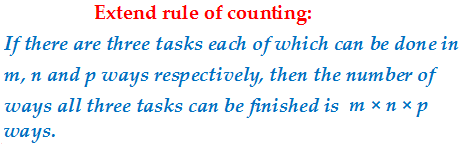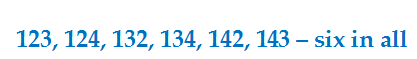## Fundamental Rule of Counting

### What is fundamental rule of counting?

Consider the following example to understand rule of counting:

Suppose your class room has two doors to enter and three to exit.

How many ways could you enter and exit your classroom?

Is it 2 + 3 ways or 2 × 3 ways?

The Rule of Counting says

It is 2 × 3 ways!

Call the two entry doors as a and b, and the three exit doors as X, Y and Z.

You have the six ways below to enter and exit from theThat’s 6 ways (3 + 3 ways, How? Think!)

Or, from the product rule - more popularly called Rule of Counting

it is 2 × 3 ways, i.e., 6 ways.

(Note that it is not 2 + 3 ways, for the rule of counting is a product rule)

So, here we have the important rule, the Rule of CountingRule of counting tells you can enter and exit class room in 2 × 3 = 6 ways.Examples (based on Rule of Counting)
1. How many numbers having 3 distinct digits can be formed with the digits 1, 2, 3, 4?

Solution:

Write three blanks, one for each digit:

_ _ _

You can write any one of 4 digits in the left most blank; any of 3 left in the middle; and only 2 in the last, i.e., one less for the next blank to keep digits distinct in the number.

To fill a blank with a digit is a task; and there are three tasks here.

Rule of counting dictates the three blanks can be filled with the four digits in:

4 × 3 × 2 = 24 ways.

Each of 24 ways is a number having 3 distinct digits.

Consider sample 3-digit numbers all beginning with 1:Likewise you can form 6 numbers with each digit in the hundred’s place.

Thus, you will make 24 numbers each having three digits.
2. How many numbers having three digits can be formed with the digits 1, 2, 3 and 4?

Solution:

How does this question differ from the previous one?

With respect to repetition of digits!

In question 1, the word “distinct” does not allow digits to repeat.

But, in this question digits can repeat as there is no word to restrict repetition.
So, you can write all 4 digits in each of the 3 blanks: _ _ _

Applying rule of counting:

the number of 3-digit numbers is 4 × 4 × 4 = 64

3. A train can ply between two stations A and B through any of 5 routes. How many routes the train can ply through between stations A and B if, to return from B to A.

1. It can take any route
2. It must take the same route
3. It cannot take the same route

Solution:
1. If the train can take any route between A and B, it has 5 routes each to
and fro. So, 5 × 5 = 25 routes.

2. Five routes are there from A to B. From B to A, only 1 route which the
train takes from A to B
3. So we have: 5 × 1 = 5

4. To return to A from B, the train cannot take one route – the route it takes
from A to B.
5 × 4 = 20
4. How many number plates can be formed with two letters first and 4 digits next, if all the letters and digits are available? Letters and digits are not allowed to repeat

Solution:
Write 6 blanks for the 2 letters and 4 digits like this: _ _ _ _ _ _.

There are 26 letters from A to Z and 10 digits from 0 to 9.

Since letters cannot repeat, write any of 26 letters in the first blank and the remaining 25 in the second blank.
From Rule of Counting, the two blanks can be filled in by 26 × 25 ways.

Now write the 10 digits for the next 4 blanks.

Without allowing digits to repeat, write 10 digits in the 3rd blank; 9 in the 4th; 8 in the 5th; 7 in the 6th blank.

Therefore, using Rule of Counting, the four blanks for the four digits can be filled in 10 × 9 × 8 × 7 ways.